# Odds and Log Odds

In the previous tutorial, you understood about logistic regression and the best fit sigmoid curve. Next, discuss Odds and Log Odds.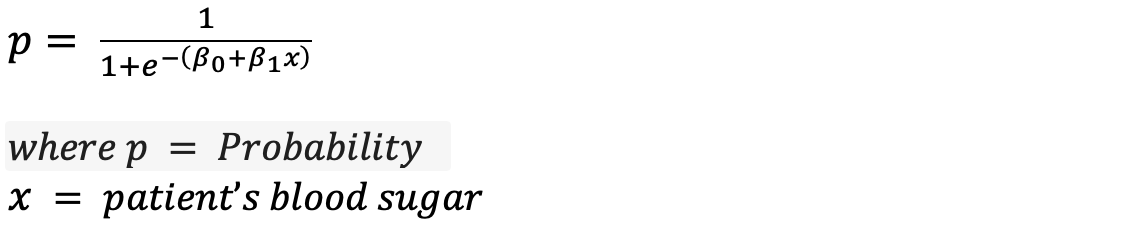##### Odds:

The relationship between x and probability is not very intuitive. Let’s modify the above equation to find an intuitive equation.

Step-1: Calculate the probability of not having blood sugar.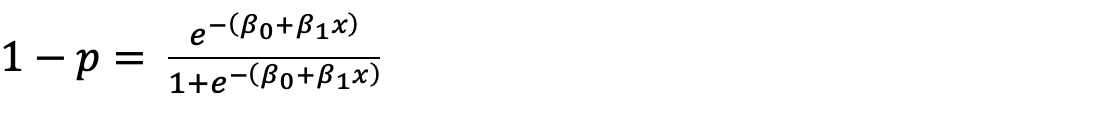Step-2: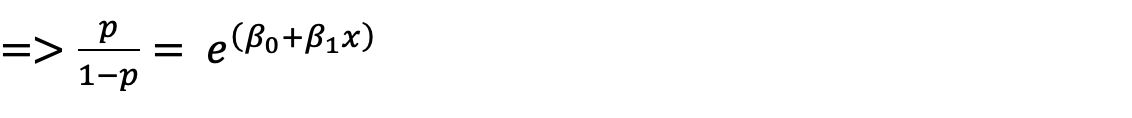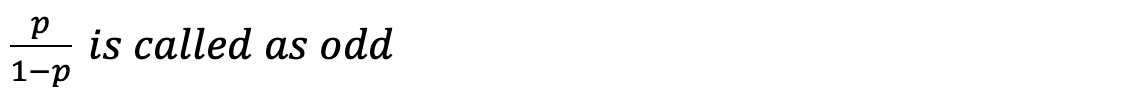Where

p = probability of having diabetes.

1-p = probability of not having diabetes.

You can interpret odd like below.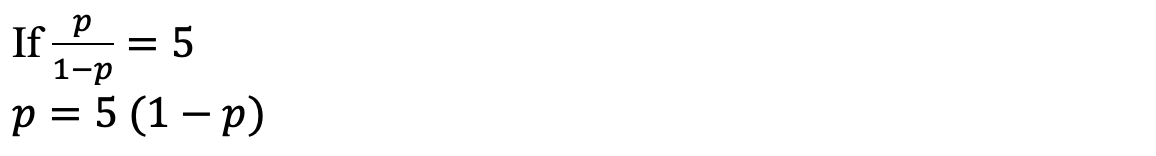This means the probability of diabetes is 5 times not having probability.

##### Log Odds:

So now that you have understood odd, let’s check out the next concept called log odds. Log odds is nothing but the logarithmic value of Odds.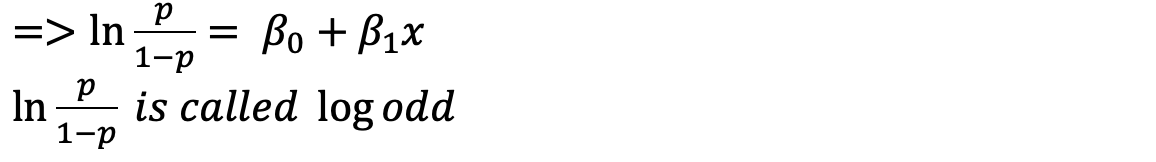Let’s use the diabetes dataset to calculate and visualize odds.

 Blood Sugar Level probability Odds Log Odds 129 0.005 0.005 -5.3 141 0.011 0.011 -4.51 160 0.034 0.036 -3.32 190 0.194 0.241 -1.42 200 0.313 0.456 -0.79 220 0.619 1.625 0.49 240 0.853 5.803 1.76 269 0.974 37.46 3.62 300 0.996 249 5.52 337 0.999 999 6.91

Upon plotting Blood sugar vs Log odds, we can observe the linear relation between blood sugar and Log Odds.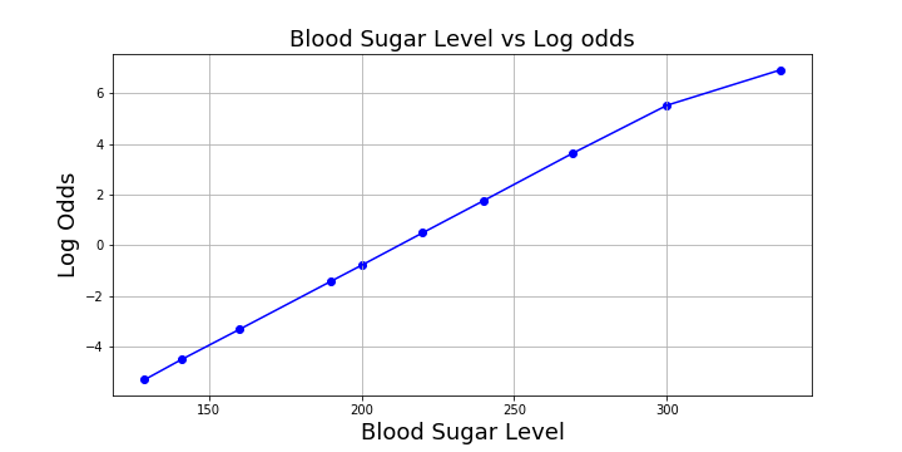Conclusion: